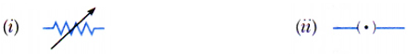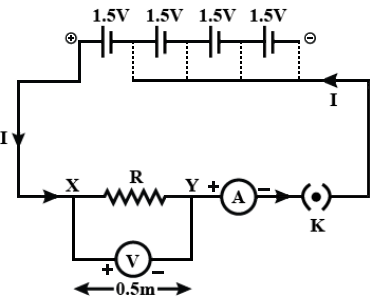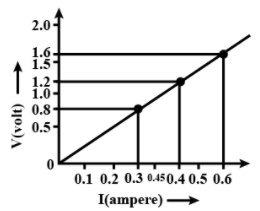• Name an instrument that measures electric current in a circuit.
Define the unit of electric current.
• What do the following symbols mean in circuit diagrams?

•• An electric circuit consisting of a 0.5 m long nichrome wire XY

• ">

# Name an instrument that measures electric current in a circuit.Define the unit of electric current.What do the following symbols mean in circuit diagrams?An electric circuit consisting of a 0.5 m long nichrome wire XY

(a) An instrument that measures electric current in a circuit is called an "ammeter". The unit of electric current is ampere (A). 1 ampere is constituted by the flow of 1 coulomb of charge through any point in an electric circuit in 1 second.

(b) The following symbols mean in circuit diagrams are:

(i) Variable resistance or Rheostat.

(ii) Plug key or switch (closed).

(c) (I)(c) (ii) Following graph was plotted between $V$ and $I$ values:At potential difference $0.8\ V$,

$\frac {V}{I}=$\frac {0.8}{0.3}=$\frac {8}{3}$

At potential difference $1.2\ V$,

​$\frac {V}{I}=$\frac {1.2}{0.45}=$\frac {8}{3}$

At potential difference $1.6\ V$,

​$\frac {V}{I}=$\frac {1.6}{0.6}=$\frac {8}{3}$​

Conclusion: If $I$ be the current through $XY$ resistor and $V$ be the potential difference across it, then the ratio $\frac {V}{I}=constant$.

$\Rightarrow V\propto I$, and Ohm's law is obeyed.

Updated on: 10-Oct-2022

31 Views